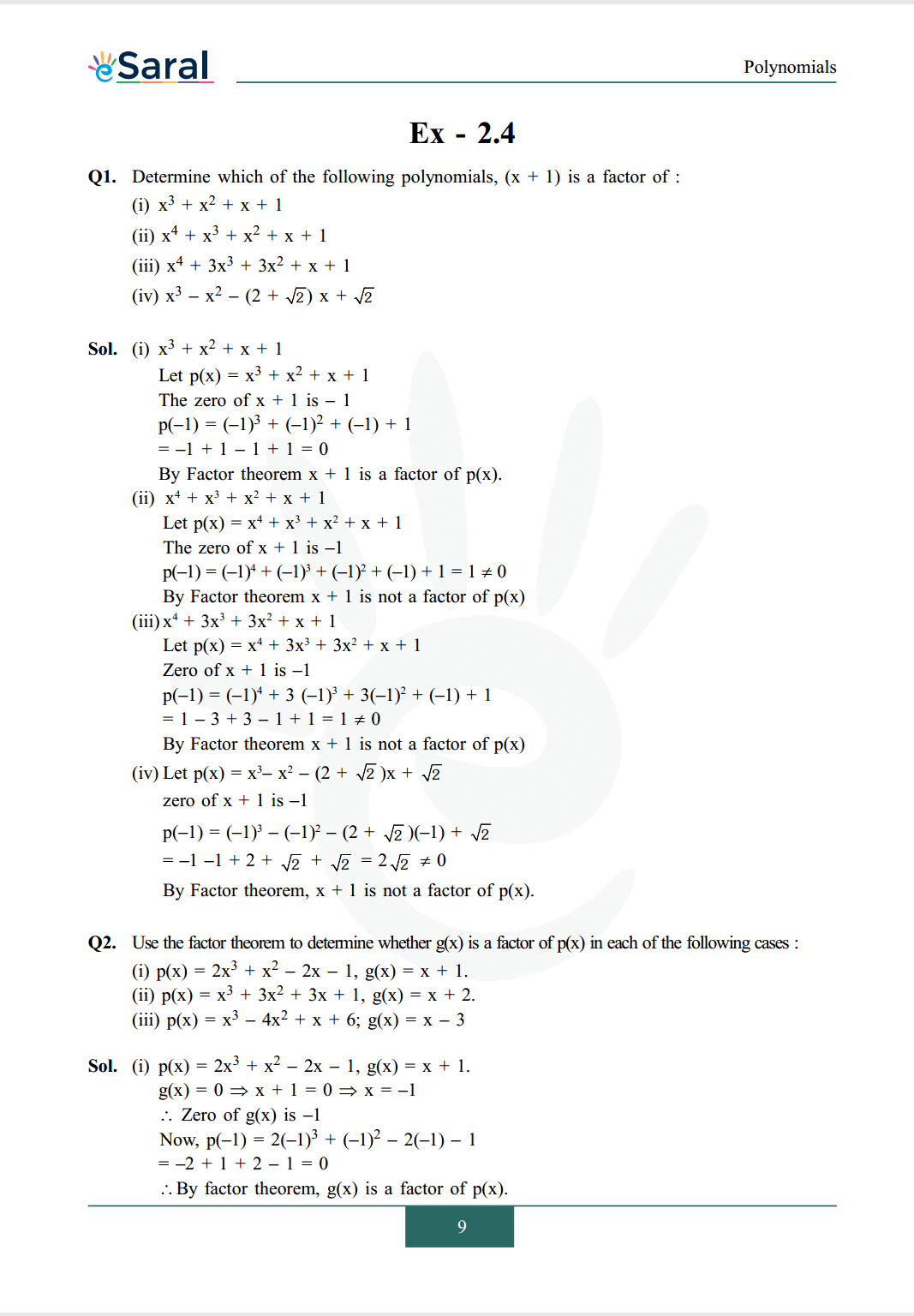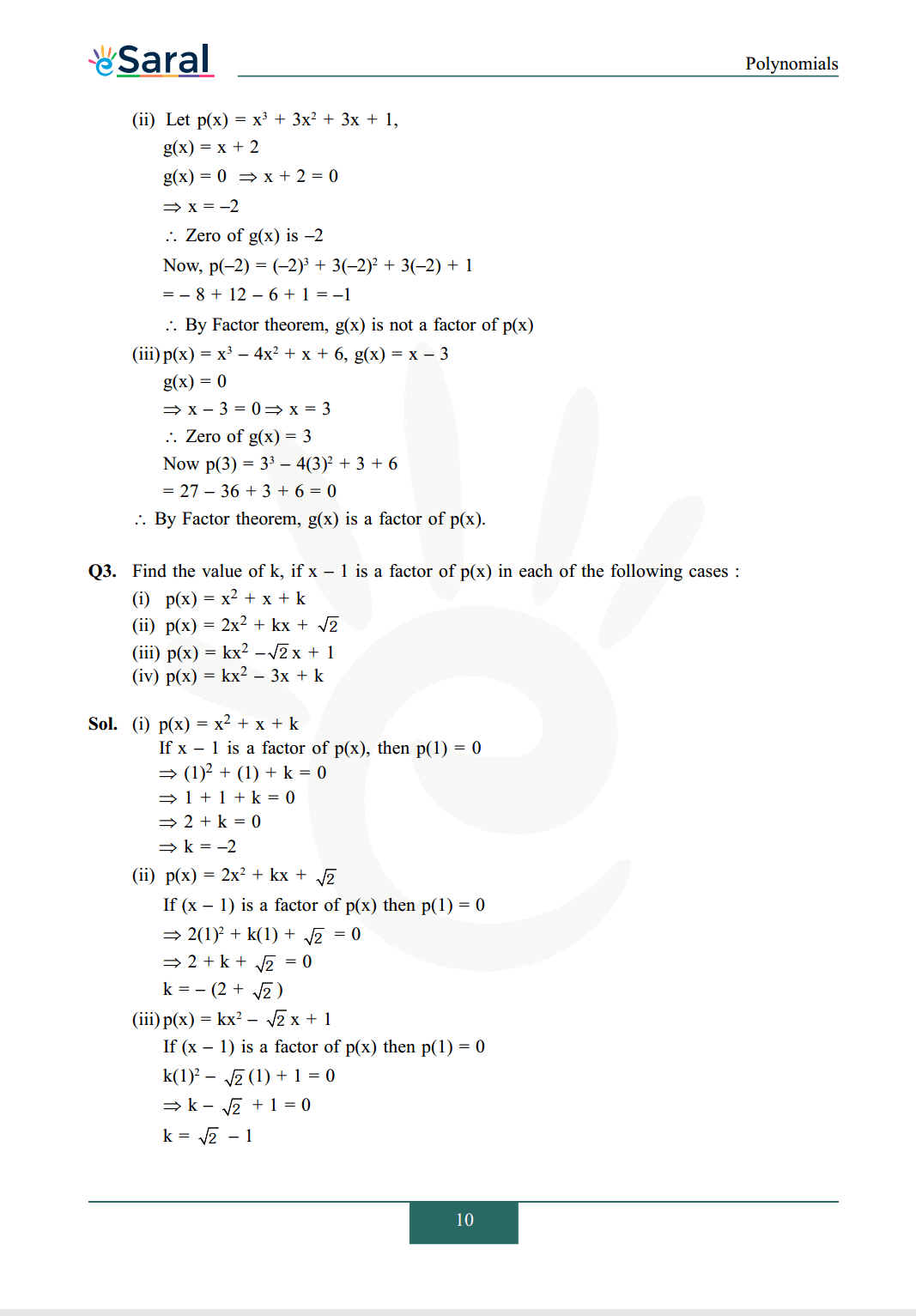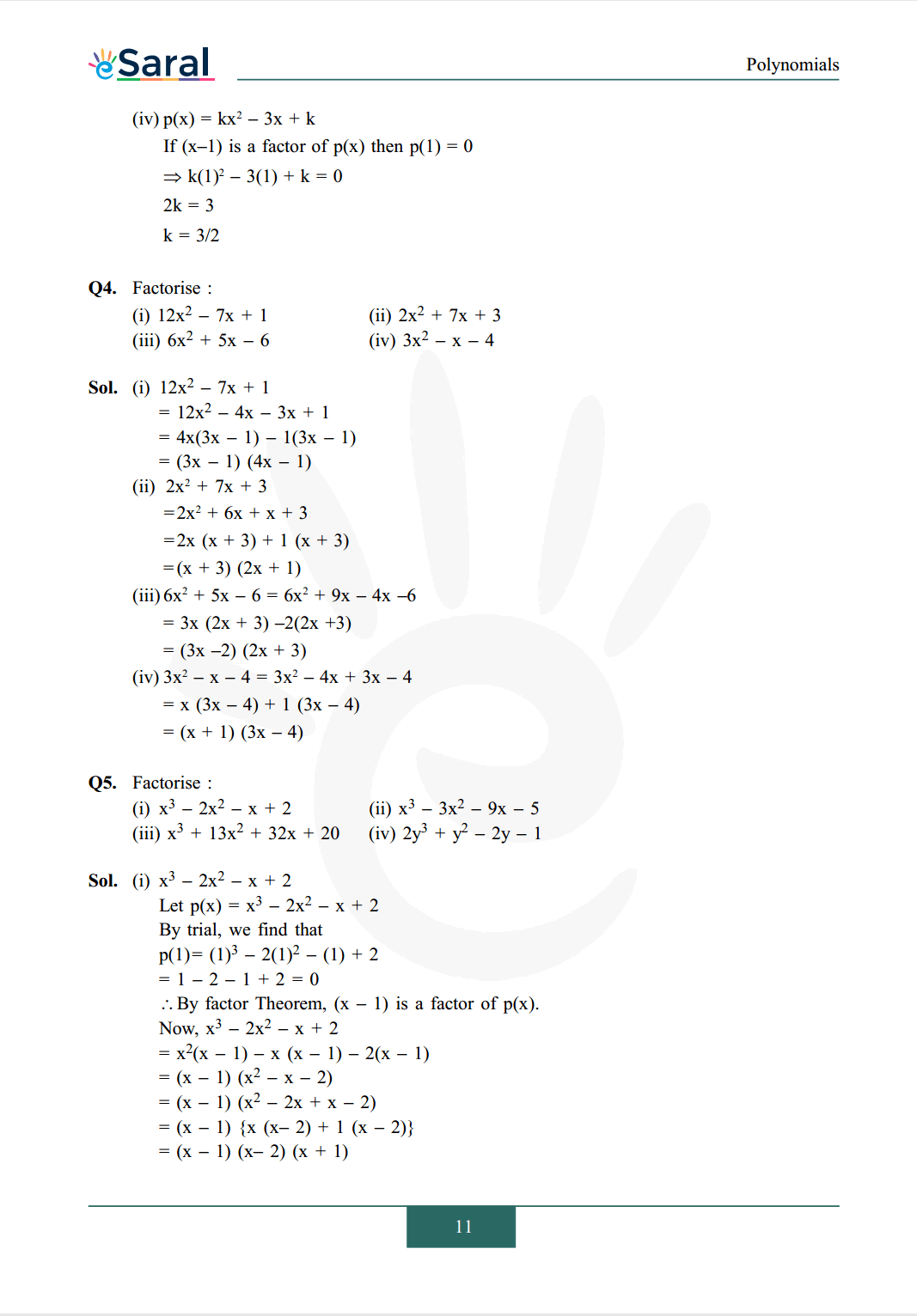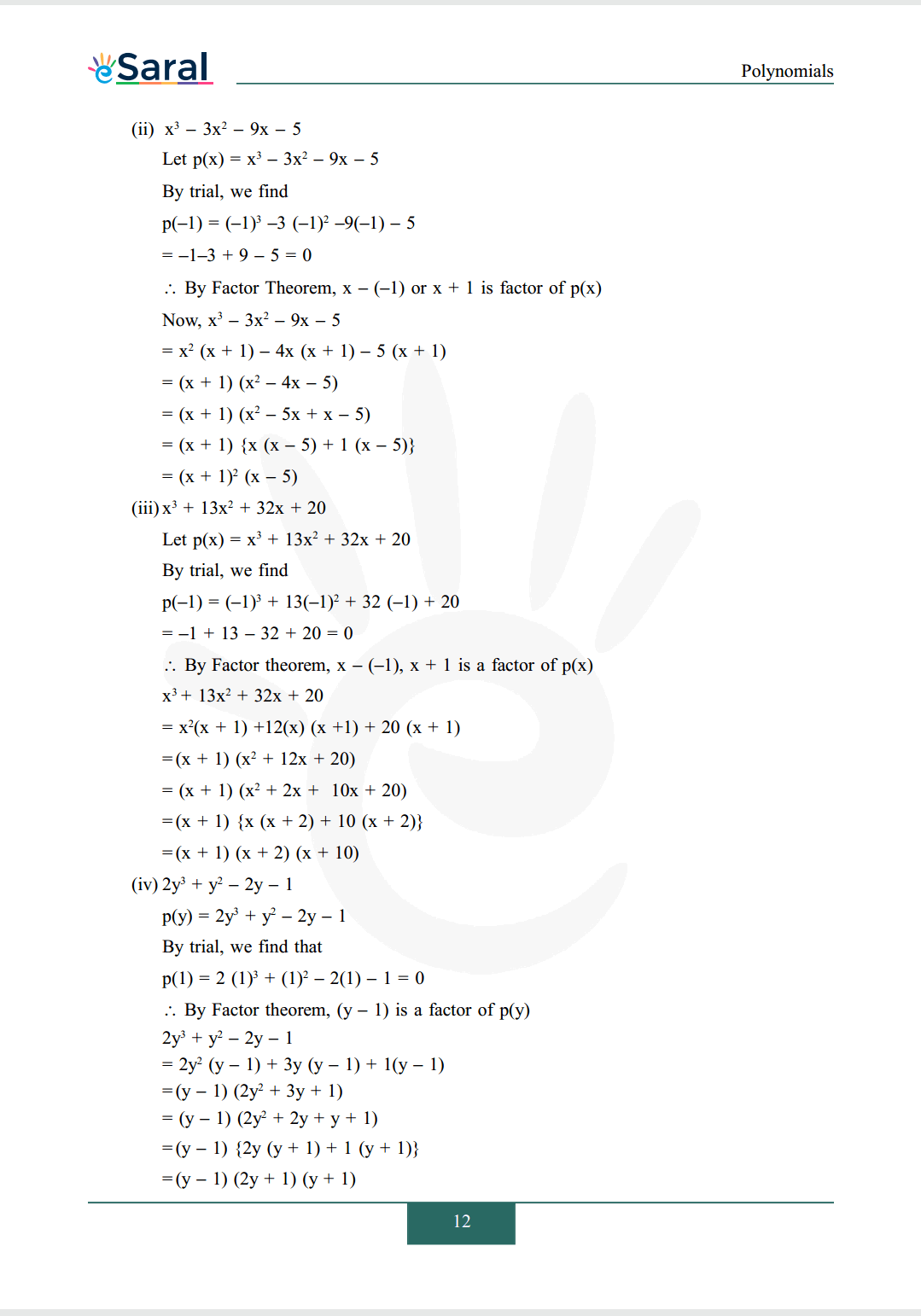Most Affordable JEE | NEET | 8,9,10 Preparation by Kota's Top IITian Doctor Faculties

# NCERT Solutions for Class 9 Maths chapter 2 Exercise 2.4 - Polynomials - Free PDF Download

Class 9`

Hey, are you a class 9 Student and Looking for Ways to Download NCERT Solutions for Class 9 Maths chapter 2 Exercise 2.4? If Yes then you are at the right place.

Here we have listed Class 9 maths chapter 2 exercise 2.4 solutions in PDF that is prepared by Kota’s top IITian’s Faculties by keeping Simplicity in mind.

If you want to score high in your class 9 Maths Exam then it is very important for you to have a good knowledge of all the important topics, so to learn and practice those topics you can use eSaral NCERT Solutions.

So, without wasting more time Let’s start.

### Download The PDF of NCERT Solutions for Class 9 Maths chapter 2 Exercise 2.4 "Polynomials"#### All Questions of Chapter 2 Exercise 2.4

Once you complete the chapter 2 then you can revise Ex. 2.4 by solving following questions

Q1. Determine which of the following polynomials, $(\mathrm{x}+1)$ is a factor of :
(i) $x^{3}+x^{2}+x+1$
(ii) $x^{4}+x^{3}+x^{2}+x+1$
(iii) $x^{4}+3 x^{3}+3 x^{2}+x+1$
(iv) $x^{3}-x^{2}-(2+\sqrt{2}) x+\sqrt{2}$

Q2. Use the factor theorem to determine whether $\mathrm{g}(\mathrm{x})$ is a factor of $\mathrm{p}(\mathrm{x})$ in each of the following cases :
(i) $\mathrm{p}(\mathrm{x})=2 \mathrm{x}^{3}+\mathrm{x}^{2}-2 \mathrm{x}-1, \mathrm{~g}(\mathrm{x})=\mathrm{x}+1$.
(ii) $\mathrm{p}(\mathrm{x})=\mathrm{x}^{3}+3 \mathrm{x}^{2}+3 \mathrm{x}+1, \mathrm{~g}(\mathrm{x})=\mathrm{x}+2$.
(iii) $\mathrm{p}(\mathrm{x})=\mathrm{x}^{3}-4 \mathrm{x}^{2}+\mathrm{x}+6 ; \mathrm{g}(\mathrm{x})=\mathrm{x}-3$

Q3. Find the value of $\mathrm{k}$, if $\mathrm{x}-1$ is a factor of $\mathrm{p}(\mathrm{x})$ in each of the following cases :
(i) $\mathrm{p}(\mathrm{x})=\mathrm{x}^{2}+\mathrm{x}+\mathrm{k}$
(ii) $\mathrm{p}(\mathrm{x})=2 \mathrm{x}^{2}+\mathrm{kx}+\sqrt{2}$
(iii) $\mathrm{p}(\mathrm{x})=\mathrm{kx}^{2}-\sqrt{2} \mathrm{x}+1$
(iv) $p(x)=k x^{2}-3 x+k$

Q4. Factorise :
(i) $12 \mathrm{x}^{2}-7 \mathrm{x}+1$
(ii) $2 \mathrm{x}^{2}+7 \mathrm{x}+3$
(iii) $6 x^{2}+5 x-6$
(iv) $3 \mathrm{x}^{2}-\mathrm{x}-4$

Q5. Factorise :
(i) $x^{3}-2 x^{2}-x+2$
(ii) $x^{3}-3 x^{2}-9 x-5$
(iii) $x^{3}+13 x^{2}+32 x+20$
(iv) $2 \mathrm{y}^{3}+\mathrm{y}^{2}-2 \mathrm{y}-1$

Class 9 NCERT Maths Book Free PDF

Class 9 NCERT Maths Exemplar Free PDF

Class 9 Maths Chapter 1 Exercise 1.1 Solutions Free PDF

Class 9 Maths Chapter 1 Exercise 1.2 Solutions Free PDF

Class 9 Maths Chapter 1 Exercise 1.3 Solutions Free PDF

Class 9 Maths Chapter 1 Exercise 1.4 Solutions Free PDF

Class 9 Maths Chapter 1 Exercise 1.5 Solutions Free PDF

If you have any Confusion related to NCERT Solutions for Class 9 Maths chapter 2 Exercise 2.4 then feel free to ask in the comments section down below.

To watch Free Learning Videos on Class 9 by Kota’s top Faculties Install the eSaral App